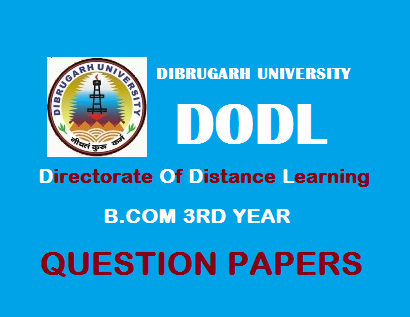## Saturday, September 02, 20172016 (August)
COMMERCE
(General)
Course: 303
(Management Accounting)
Full Marks: 90

Time: 3 hours

The figures in the margin indicate full marks for the questions

1. (a) Fill in the blanks: 1x5=5
1. Management Accounting is concerned with accounting information that is useful to ____.
2. Cash receipt from issue of shares is a ____ activity.
3. Contribution is the difference between sales and ____.
4. Sales budget is a ____ budget.
5. The difference between actual cost and standard cost is ____.
(b) Write ‘True’ or ‘False’: 1x5=5

1. The primary goal of management accounting is to provide information for planning, decision making etc.
2. Profit is the life-blood of every business concern.
3. Marginal Cost = Total Cost – Variable Cost.
4. Flexible Budget is a dynamic budget.
5. Variance is a tool of cost control and cost reduction.
2. Write short notes on: 4x5=20
1. Limitations of Management Accounting.
2. Limitations of Fund Flow Statement.
3. P/v Ratio.
4. Cash Budget.
5. Material Price Variance.
3. (a) “Management Accounting is concerned with accounting information that is useful to management.” Explain this statement and discuss clearly the differences between Management Accounting and Financial Accounting. 4+8=12
Or
(b) Define Management Accounting. Discuss its functions and limitations. 3+5+4=12
4. (a) Explain the meaning, importance and objectives of Fund Flow Statement. 3+4+5=12
Or
(b) The following are the Balance Sheets of Blue Sky Company Ltd. for the year 2014-15 and 2015-16:
Balance Sheets
 Liabilities 2014-15Rs. 2015-16Rs. Assets 2014-15Rs. 2015-16Rs. Share Capital shares of Rs. 10 each Profit & Loss A/c 10% Debentures Creditors 3,50,000 50,400 60,000 51,600 3,70,000 52,800 30,000 59,200 Land StocksGoodwill Cash Debtors 1,00,0002,46,00050,00045,00071,000 1,50,0002,13,50025,00039,00084,500 5,12,000 5,12,000 5,12,000 5,12,000

1. Dividend paid during the year Rs. 17,500.
2. Land was revalued during the year at 1,50,000 and the profit on revaluation transferred to Profit & Loss A/c.
From the above information, prepare a Cash Flow Statement for the year ended 31st March, 2015-16. 12
5. (a) From the following particulars, calculate: 3+4+5=12
1. P/v Ratio.
2. Profit when sales are Rs. 20,000.
3. New Break Even Point if selling price is reduced by 20%.
Fixed Expenses Rs. 4,000/-
Break Even Point…. Rs. 10,000/-
Or
(b) “The technique of marginal costing is a valuable aid to management” – Discuss. 12
6. (a) From the production of 10,000 watches, the following were the budgeted expenses:
 Per Unit (Rs.) Direct Material Direct Labour Variable Overheads Fixed Expenses (Rs. 1,50,000)Variable Overheads (Direct)Selling Expenses (10% fixed)Administration Expenses Rs. 50,000 in all levels of productionDistribution Expenses (20% Fixed) 6030251551555 Total Cost 160

Prepare a budget for the production of 6,000 and 8,000 watches. 12
Or
(b) Explain the meaning and objectives of Sales Budget. Discuss briefly about the preparation of Sales Budget. 3+4+5=12

7. (a) It has been mentioned that the cost standards for materials consumption are 20 Kg @ Rs. 5 per Kg. Compute the variances when actual are: 3x4=12
1. 24 Kg. at Rs. 5 per Kg.
2. 20 Kg. at Rs. 6 per Kg.
3. 24 Kg. at Rs. 6 per Kg.
4. 18 Kg. for a total cost of Rs. 180
Or
(b) What do you mean by ‘Standard Costing’? What are its objectives? Distinguish between ‘Standard Cost’ and Estimated Cost’. 3+4+5=12